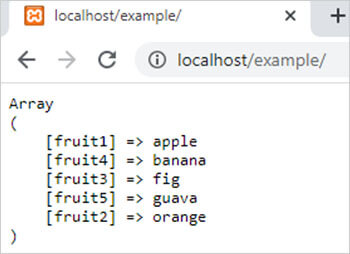asort() function in PHP || PHP asort function# asort() function in php

1591

PHP asort() function is used to sort an associative PHP array in ascending order. It is an inbuilt function of PHP. This function sorts the array according to the values.

Syntax

asort(\$array,\$type_of_sorting);

here,

1) \$array is a PHP array which we want to sort. It is mandatory.

2) \$type_of_sorting is a numeric value that decides the type of comparing of array elements. It is optional. It has 6 possible values that specify 6 possible sorting orders.

• SORT_REGULAR: If a value is 0 then the function compares array elements normally.
• SORT_NUMERIC: If a value is 1 then the function compares array elements as a numeric value.
• SORT_STRING: If a value is 2 then the function compares array elements as a string.
• SORT_LOCALE_STRING: If a value is 3 then this function compares array elements as a string according to the current locale.
• SORT_NATURAL: If a value is 4 then the function compares array elements as a string with the use of natural ordering.
• SORT_FLAG_CASE: If a value is 5 then this function compares array elements according to value.

Example:

```<?php
\$arr=array("fruit1"=>"apple","fruit2"=>"orange","fruit3"=>"fig","fruit4"=>"banana","fruit5"=>"guava");
asort(\$arr);
echo "<pre>";
print_r(\$arr);
?>```

Output:Share:## Big Hosting Days

Upto 75@ OFF on Web Hosting

## Get a .COM for just \$6.98

Secure Domain for a Mini Price